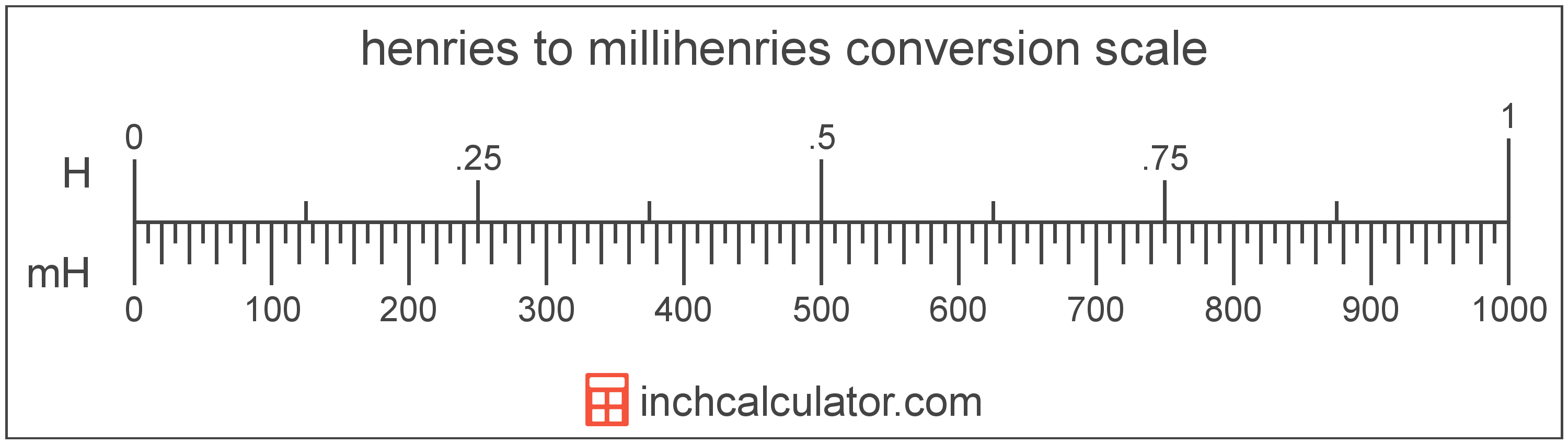# Henries to Millihenries Converter

Enter the electrical inductance in henries below to get the value converted to millihenries.

Results in Millihenries:1 H = 1,000 mH

Do you want to convert millihenries to henries?

## How to Convert Henries to Millihenries

To convert a measurement in henries to a measurement in millihenries, multiply the electrical inductance by the following conversion ratio: 1,000 millihenries/henry.

Since one henry is equal to 1,000 millihenries, you can use this simple formula to convert:

millihenries = henries × 1,000

The electrical inductance in millihenries is equal to the electrical inductance in henries multiplied by 1,000.

For example, here's how to convert 5 henries to millihenries using the formula above.
millihenries = (5 H × 1,000) = 5,000 mH### How Many Millihenries Are in a Henry?

There are 1,000 millihenries in a henry, which is why we use this value in the formula above.

1 H = 1,000 mH

## What is a Henry?

One henry is equal to the inductance of a conductor in which there is one volt of electromotive force when the current through the conductor is increased by one ampere per second.

The henry is the SI derived unit for electrical inductance in the metric system. Henries can be abbreviated as H; for example, 1 henry can be written as 1 H.

The National Institute of Standards and Technology recommends using "henries" as the plural form of the unit, however the usage of "henrys" is also common.

## What is a Millihenry?

One millihenry is equal to 1/1,000 of a henry, which is the inductance of a conductor with one volt of electromotive force when the current is increased by one ampere per second.

The millihenry is a multiple of the henry, which is the SI derived unit for electrical inductance. In the metric system, "milli" is the prefix for thousandths, or 10-3. Millihenries can be abbreviated as mH; for example, 1 millihenry can be written as 1 mH.

## Henry to Millihenry Conversion Table

Table showing various henry measurements converted to millihenries.
Henries Millihenries
0.001 H 1 mH
0.002 H 2 mH
0.003 H 3 mH
0.004 H 4 mH
0.005 H 5 mH
0.006 H 6 mH
0.007 H 7 mH
0.008 H 8 mH
0.009 H 9 mH
0.01 H 10 mH
0.02 H 20 mH
0.03 H 30 mH
0.04 H 40 mH
0.05 H 50 mH
0.06 H 60 mH
0.07 H 70 mH
0.08 H 80 mH
0.09 H 90 mH
0.1 H 100 mH
0.2 H 200 mH
0.3 H 300 mH
0.4 H 400 mH
0.5 H 500 mH
0.6 H 600 mH
0.7 H 700 mH
0.8 H 800 mH
0.9 H 900 mH
1 H 1,000 mH

## References

1. International Bureau of Weights and Measures, The International System of Units, 9th Edition, 2019, https://www.bipm.org/documents/20126/41483022/SI-Brochure-9-EN.pdf
2. Ambler Thompson and Barry N. Taylor, Guide for the Use of the International System of Units (SI), National Institute of Standards and Technology, https://physics.nist.gov/cuu/pdf/sp811.pdf